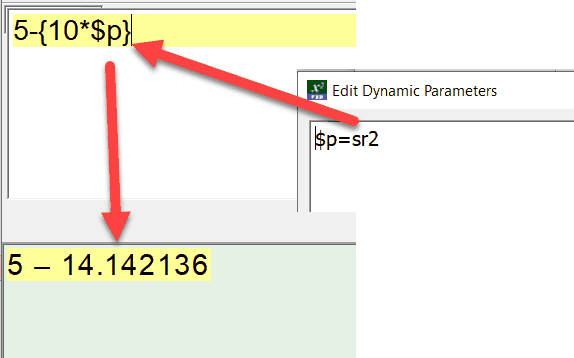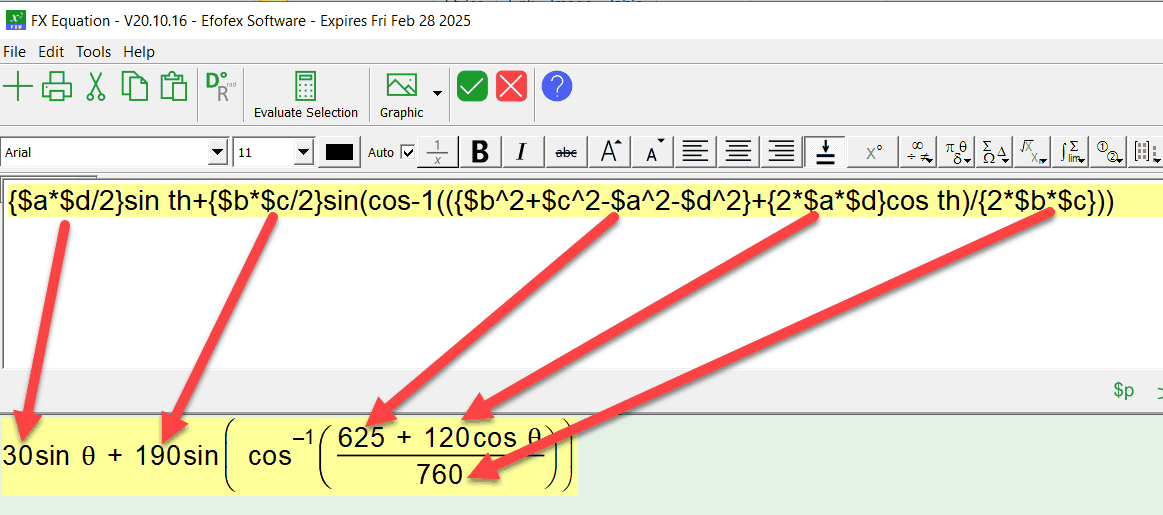﻿ Using Parameters In Calculations

# Using Parameters In Calculations

Top  Previous  Next
 Any parameters you include in a calculation will be resolved prior to the calculation being performed. For example, we have defined \$p to be the square root of 2 in the next example.One equation can contain multiple inline calculations. Combined with parameters, you can build complicated equations with FX Equation performing most of the calculations automatically.